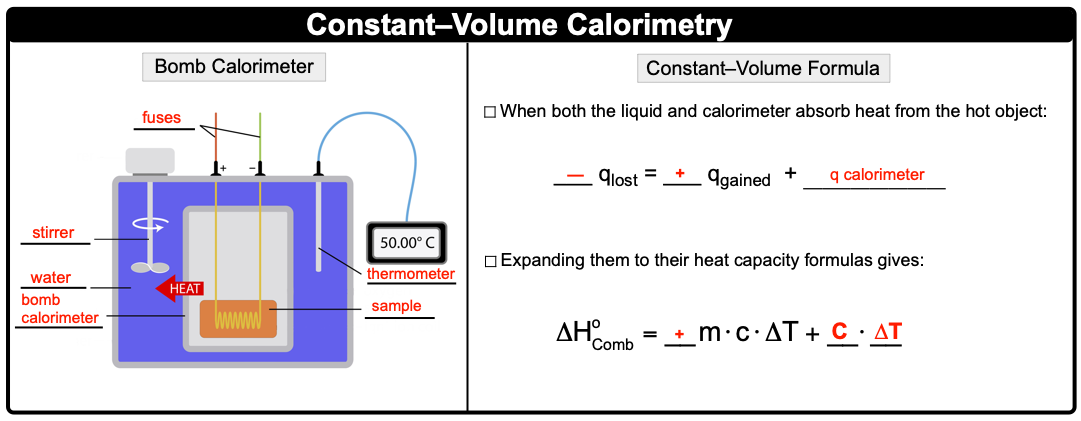Clutch Prep is now a part of Pearson
Ch.6 - Thermochemistry WorksheetSee all chapters

# Constant-Volume Calorimetry

See all sections
Sections
Nature of Energy
Kinetic & Potential Energy
First Law of Thermodynamics
Internal Energy
Endothermic & Exothermic Reactions
Heat Capacity
Constant-Pressure Calorimetry
Constant-Volume Calorimetry
Thermal Equilibrium
Thermochemical Equations
Formation Equations
Enthalpy of Formation
Hess's Law

Constant-Volume Calorimetry uses a bomb calorimeter and a combustion reaction to determine its enthalpy of reaction.

###### Constant-Volume Calorimetry

Concept #1: Heat of Combustion

Heat of Combustion is the amount of heat released when 1 mole of substance is burned or combusted in a Bomb Calorimeter.

Example #1: Which of the following statements is true about the combustion of propane?

Concept #2: Bomb CalorimeterExample #2: The heat capacity of a bomb calorimeter was determined by burning 12.13 g ethane (heat of combustion = 1560 kJ/mol) in the bomb. If the temperature changed by 15.2 ºC, what is the heat capacity of the bomb?